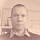# Data Science: Computation of Eigenvectors — Deflation Method.

This story is part of my Data Science series.

In our previous story (see here), we have looked at a procedure to compute the in absolute terms highest eigenvalue of a finite dimensional endomorphism — a `(n,n)`-matrix. For applications like the principle component analysis we need all eigenvalues, or at least a large part, to make satisfying conclusions.

This story is devoted to look at some relatively easy approach that is applicable for symmetric matrices with distinct eigenvalues. But let me note, this is by far not the best-known technique - there exists many more. The entire area of eigenvalue solvers is an ongoing subject of sophisticated research!

## Implementation:

We are going make the implementation proceeded what we have started in the previous story (see here). The dependency in `Cargo.toml` will stay the same.## An Introduction into RustFirst of all we need a way to extend a given vector in some `R^n` to a basis of the latter. This can be easily done like follows: